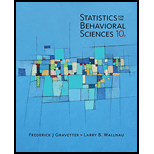# Two separate samples receive different treatments. After treatment, the first sample has n = 9 with S S = 462 , and the second has n = 7 with S S = 420 . a. Compute the pooled variance for the two samples. b. Calculate the estimated standard error for the sample mean difference. c. If the sample mean difference is 10 points, is this enough to reject the null hypothesis using a two-tailed test with α = .05 ?### Statistics for The Behavioral Scie...

10th Edition
Frederick J Gravetter + 1 other
Publisher: Cengage Learning
ISBN: 9781305504912### Statistics for The Behavioral Scie...

10th Edition
Frederick J Gravetter + 1 other
Publisher: Cengage Learning
ISBN: 9781305504912

#### Solutions

Chapter
Section
Chapter 10, Problem 6P
Textbook Problem

## Expert Solution

### Want to see the full answer?

Check out a sample textbook solution.See solution

### Want to see this answer and more?

Experts are waiting 24/7 to provide step-by-step solutions in as fast as 30 minutes!*

See Solution

*Response times vary by subject and question complexity. Median response time is 34 minutes and may be longer for new subjects.

Find more solutions based on key concepts
Show solutions
Find the average value of the function f(t) = sec2t on the interval [0, /4].

Single Variable Calculus: Early Transcendentals, Volume I

Expand each expression in Exercises 122. (2y+3)(y+5)

Finite Mathematics and Applied Calculus (MindTap Course List)

g(x)=2(x1)5/2

Applied Calculus for the Managerial, Life, and Social Sciences: A Brief Approach

Sometimes, Always, or Never: limxax=0.

Study Guide for Stewart's Single Variable Calculus: Early Transcendentals, 8th

Identify the two characteristics needed for a research study to qualify as an experiment.

Research Methods for the Behavioral Sciences (MindTap Course List)

Reminder Round all answers to two decimal places unless otherwise indicated. Total Revenue and ProfitThis is a ...

Functions and Change: A Modeling Approach to College Algebra (MindTap Course List)

Consider the following hypothesis test. The following data are from matched samples taken from two populations...

Modern Business Statistics with Microsoft Office Excel (with XLSTAT Education Edition Printed Access Card) (MindTap Course List)

The function y = sin x is an explicit solution of the first-order differential equation dydx=1y2. Find an inter...

A First Course in Differential Equations with Modeling Applications (MindTap Course List)